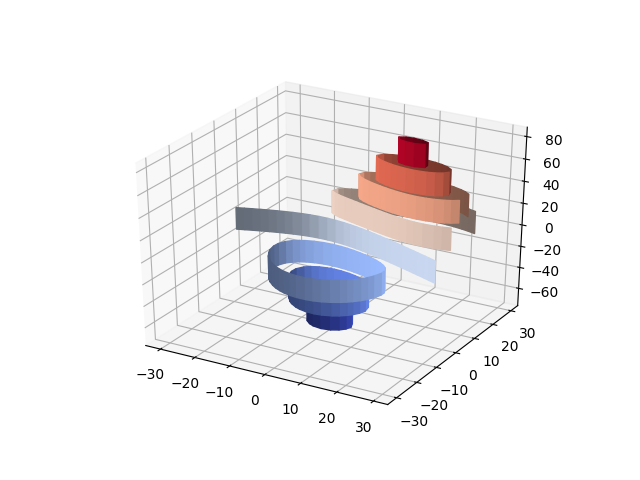Travis-CI:# Demonstrates plotting contour (level) curves in 3D using the extend3d option¶

This modification of the contour3d_demo example uses extend3d=True to extend the curves vertically into ‘ribbons’.```from mpl_toolkits.mplot3d import axes3d
import matplotlib.pyplot as plt
from matplotlib import cm

fig = plt.figure()
ax = fig.gca(projection='3d')
X, Y, Z = axes3d.get_test_data(0.05)

cset = ax.contour(X, Y, Z, extend3d=True, cmap=cm.coolwarm)

ax.clabel(cset, fontsize=9, inline=1)

plt.show()
```

Total running time of the script: ( 0 minutes 0.045 seconds)

Gallery generated by Sphinx-Gallery